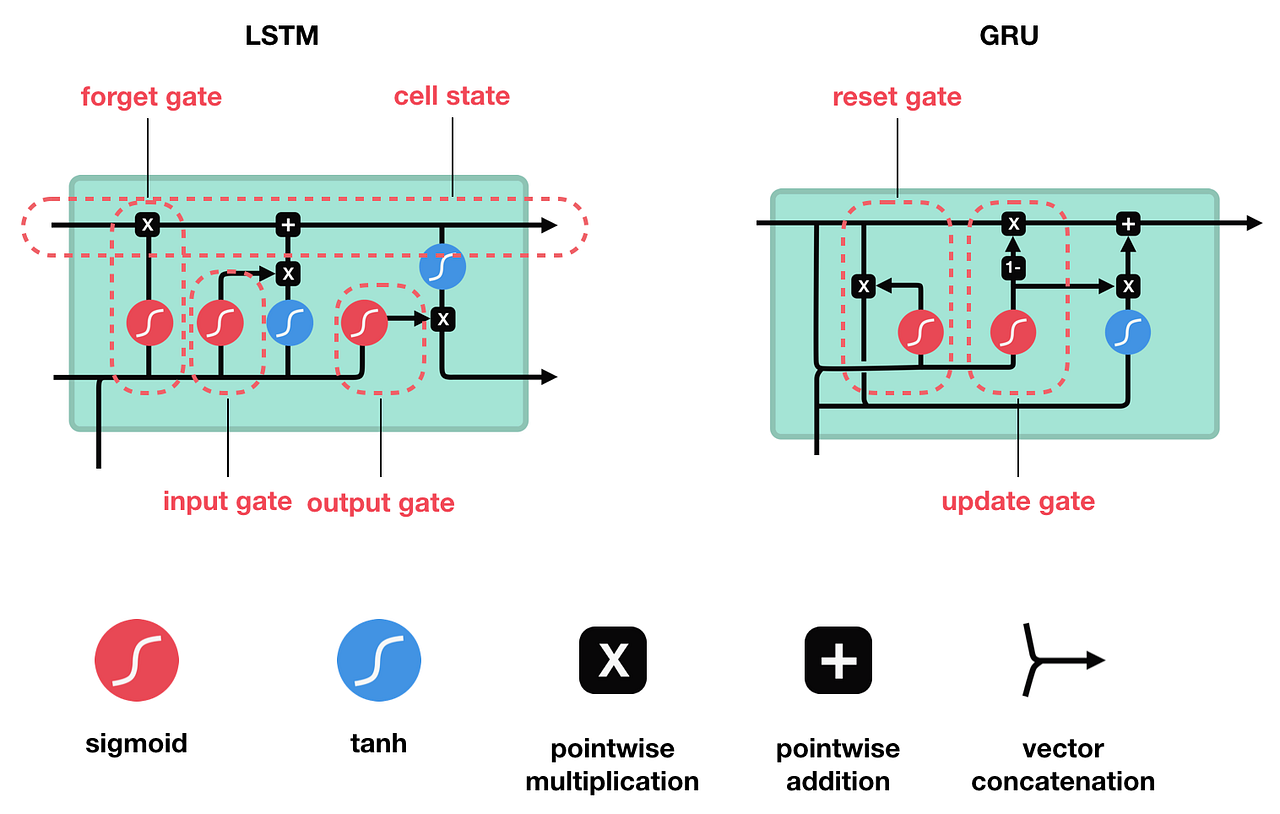# What's the difference between LSTM and GRU?

I have been reading about LSTMs and GRUs, which are recurrent neural networks (RNNs). The difference between the two is the number and specific type of gates that they have. The GRU has an update gate, which has a similar role to the role of the input and forget gates in the LSTM.

Here's a diagram that illustrates both units (or RNNs).With respect to the vanilla RNN, the LSTM has more "knobs" or parameters. So, why do we make use of the GRU, when we clearly have more control over the neural network through the LSTM model?

Here are two more specific questions.

1. When would one use Long Short-Term Memory (LSTM) over Gated Recurrent Units (GRU)?

On the same problems, sometimes GRU is better, sometimes LSTM.

In short, having more parameters (more "knobs") is not always a good thing. The training process needs to learn those parameters. There is a higher chance of over-fitting, amongst other problems.

The parameters are assigned specific roles inside either GRU or LSTM, so if that role is less important for a specific learning challenge, then it can be wasteful or even counter-productive to have the system attempt to learn values for them.

The only way to find out if LSTM is better than GRU on a problem is a hyperparameter search. Unfortunately, you cannot simply swap one for the other, and test that, because the number of cells that optimises a LSTM solution will be different to the number that optimises a GRU.

When would one use Long Short-Term Memory (LSTM) over Gated Recurrent Units (GRU)?

When it proves better, experimentally. In some problem domains, this may be established and you can check. However, in other problem domains if either GRU or LSTM works well enough to solve a problem (and the superiority of either LSTM or GRU is not the main point of the work), then it may not so clear.

• Overall, this answer seems to be correct, but I think this answer would improve considerably if you provided some concrete examples of applications/problems where GRU was used over LSTM (or vice-versa) and why it was used. This would at least provide some more "proof" that the preference for one or the other may be context-dependent.
– nbro
May 23, 2020 at 0:07